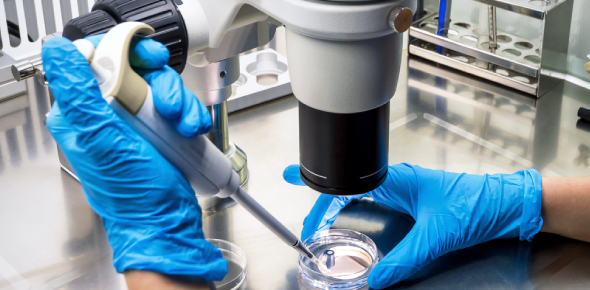# The Candid Test On Microbiology

68 Questions | Attempts: 162SettingsMicrobiology includes fundamental research on the biochemistry, physiology, cell biology, ecology, evolution and clinical aspects of microorganisms, including the host response to these agents. One gets to understand how the cells in living things operate. Have you just covered chapter 9 on biology? Take up the candid test on biology below and refresh your memory.

• 1.
__________ reactions capture energy from the organisms' energy source.
• 2.
_________ is the total of all chemical reactions occurring in the cell.
• 3.
________ reactions build new organic molecules from smaller inorganic and organic compounds.
• 4.
Cells carry out three major types of work; which of the following involves the synthesis of macromolecules as well as the breakdown of substances for their energy?
• A.

Chemical work

• B.

Transport work

• C.

Mechanical work

• D.

None of the above

• 5.
Cells carry out three major types of work; which of the following involves nutrient uptake and waste elimination?
• A.

Chemical work

• B.

Transport work

• C.

Mechanical work

• D.

None of the above

• 6.
Cells carry out three major types of work; which of the following involves energy for cell motility and the movement of structures within cells?
• A.

Chemical work

• B.

Transport work

• C.

Mechanical work

• D.

None of the above

• 7.
In thermodynamic studies, energy changes are analyzed in a collection of matter called a __________. All other matter in the universe is called the __________. (answer, answer)
• 8.
__________ is the science that analyzes energy changes in a collection of matter.
• 9.
Energy can be redistributed within a collection of matter (called a system) or can be redistributed between the system and its surroundings.
• A.

True

• B.

False

• 10.
__________ is a measure of the randomness or disorder of a system.
• A.

Entropy

• B.

Enthalpy

• C.

Free energy

• D.

Synergy

• 11.
The __________ law of thermodynamics states that physical and chemical processes occur in such a way that randomness (disorder) increases to a maximum.
• A.

First

• B.

Second

• C.

Third

• D.

Fourth

• 12.
The __________ law of thermodynamics states that energy can be neither created nor destroyed.
• A.

First

• B.

Second

• C.

Third

• D.

Fourth

• 13.
The amount of heat energy needed to raise 1 gram of water from 14.5C to 15.5C is called a(n)
• A.

Joule

• B.

Calorie

• C.

Erg

• D.

Thermal unit

• 14.
A reaction in which the forward rate is equal to the reverse rate is said to be at __________.
• 15.
The numerical value of the free energy change indicates how fast a reaction will reach equilibrium.
• A.

True

• B.

False

• 16.
__________ is the total energy change that accompanies a chemical reaction.
• A.

Entropy

• B.

Enthalpy

• C.

Free energy

• D.

Synergy

• 17.
For the reaction A + B C + D, the equilibrium constant (Keq) is defined as
• A.

[A][B]/[C][D]

• B.

[C][D]/[A][B]

• C.

[A][D]/[B][C]

• D.

[B][C]/[A][D]

• 18.
The change in __________ is the amount of energy in a system that is available to do work.
• A.

Entropy

• B.

Enthalpy

• C.

Free energy

• D.

Synergy

• 19.
The most commonly used practical form of energy used in cells is adenosine diphosphate.
• A.

True

• B.

False

• 20.
A reaction that releases energy is __________.
• A.

Exergonic

• B.

Endergonic

• C.

Impossible

• D.

None of the choices

• 21.
Some endergonic reactions can be made to proceed forward if they are coupled to hydrolysis of one or more of the phosphates of ATP.
• A.

True

• B.

False

• 22.
Cells must efficiently transfer energy from their energy-trapping systems to the systems actually carrying out work and also use various metabolic processes to replace the energy used in doing work. This is called the __________. (2 words)
• 23.
In order for the cell to be able to input energy into necessary endergonic reactions, energygenerating processes such as photosynthesis, fermentation, and respiration are used to produce __________.
• 24.
An chemical reaction that requires an input of energy in order to proceed is __________.
• A.

Exergonic

• B.

Endergonic

• C.

Impossible

• D.

None of the choices

• 25.
The __________ is the electron acceptor in a redox reaction.
• A.

Reductant

• B.

Oxidant

• C.

Enzyme

• D.

Product

## Related TopicsBack to top
×

Wait!
Here's an interesting quiz for you.